#### Vol. 15, No. 2, 1965

 Download this articleFor screen For printingRecent Issues Vol. 304: 1 Vol. 303: 1  2 Vol. 302: 1  2 Vol. 301: 1  2 Vol. 300: 1  2 Vol. 299: 1  2 Vol. 298: 1  2 Vol. 297: 1  2Online Archive Volume: Issue:The Journal Editorial Board Subscriptions Officers Special Issues Submission Guidelines Submission Form Contacts ISSN: 1945-5844 (e-only) ISSN: 0030-8730 (print) Author Index To Appear Other MSP Journals
Minimal Gerschgorin sets

### Richard Steven Varga

Vol. 15 (1965), No. 2, 719–729
##### Abstract

If A = (ai,j) is a fixed n × n complex matrix, then it is well known that the Gerschgorin disks Gi in the complex plane, defined by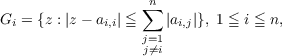(1)

are such that each eigenvalue of A lies in at least one disk, and, consequently, the union of these disks,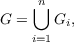(2)

which we call the Gerschgorin set, contains all the eigenvalues of A. It is however clear from (1) that the radii of these Gerschgorin disks depend only on the moduli of the off-diagonal entries of A. Thus, if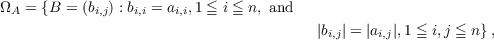(3)
then it is clear that the Gerschgorin set G contains all the eigenvalues of each n×n matrix B in ΩA. It is natural to ask how far-reaching this elementary theory is in bounding the eigenvalues of ΩA.

To extend the above results slightly, let x > 0 be any vector with positive components, and let X(x) diag(x1,x2,,xn). Applying the above results to X1(x)AX(x) shows that if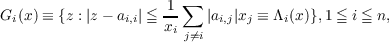(1’)

then the associated Gerschgorin set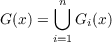(2’)

again contains all the eigenvalues of each B ΩA for every x > 0. Thus, the closed bounded set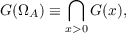(4)

which we call the minimal Gerschgorin set, also contains all the eigenvalues of each B ΩA.

One of the major results in this paper is that each boundary point of GA) is an eigenvalue of some matrix B in ΩA.

Primary: 15.25
##### Milestones